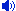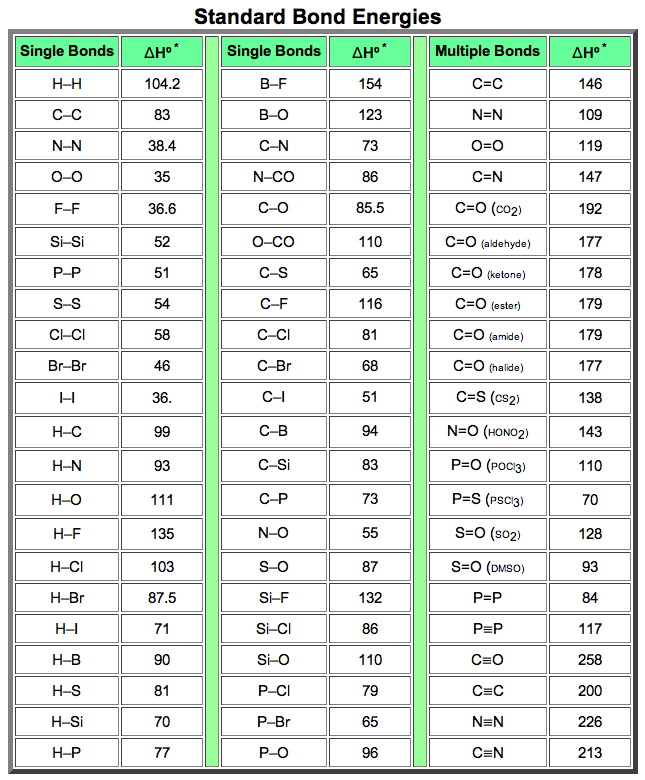Bond Energy

##Bond EnergySince reactions of organic compounds involve the making and breaking of bonds, the strength of bonds, or their resistance to breaking, becomes an important consideration. For example, the chlorination of methane, discussed previously, was induced by breaking a relatively weak Cl-Cl covalent bond.Bond energy is the energy required to break a covalent bond homolytically (into neutral fragments). Bond energies are commonly given in units of kcal/mol or kJ/mol, and are generally called bond dissociation energies when given for specific bonds, or average bond energies when summarized for a given type of bond over many kinds of compounds.Tables of bond energies may be found in most text books and handbooks. The following table is a collection of average bond energies for a variety of common bonds. Such average values are often referred to as standard bond energies, and are given here in units of kcal/mole.The SI unit of energy is the joule, symbol J. To convert kilocalories into kilojoules multiply by 4.184. A useful site for unit conversions may be reached by Clicking Here.* Average Bond Dissociation Enthalpies in kcal mole-1Some useful and interesting conclusions may be drawn from this table. First, a single bond between two given atoms is weaker than a double bond, which in turn is weaker than a triple bond.Second, hydrogen forms relatively strong bonds (90 to 110 kcal) to the common elements found in organic compounds (C, N & O).Third, with the exception of carbon and hydrogen, single bonds between atoms of the same element are relatively weak (35 to 64 kcal).Indeed, the fact that carbon forms relatively strong bonds to itself as well as to nitrogen, oxygen and hydrogen is a primary factor accounting for the very large number of stable organic compounds.

Virtual Textbookの目次へ